# Percentages + cylinder - math problems

#### Number of problems found: 15

• CylinderIn a 1-meter diameter cylinder is 1413 liters of water, which is 60% of the cylinder. Calculate the cylinder height in meters, do not write the units. The resulting value round and write as an integer.
• Cone in cylinder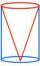The cylinder is inscribed cone. Determine the ratio of the volume of cone and cylinder. The ratio express as a decimal number and as percentage.
• LatheCalculate the percentage of waste if the cube with 53 cm long edge is lathed to cylinder with a maximum volume.
• LatheFrom the cube of edge, 37 cm was a lathed maximum cylinder. What percentage of the cube is left as waste after lathed?
• Water reservoir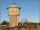The reservoir shape of a cylinder having a diameter 8 m and a height 2.8 m. The device indicates that the reservoir contains 800 hectoliters of water. Calculate how much % of volume is used.
• Fuel economyHow many kilometers is sufficient petrol in the cylinder fuel tank with a diameter 40 cm and the base of tank length 1 m, when it is filled to 60% and if the car consume 15 liters per 100 km?
• HectolitersHow many hectoliters of water is in garden barrel with 90 cm diameter and a height of 1.3 m, if it is filled to 80% of its capacity?
• Gutter pipe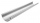How many m² of sheet metal is required to produce a 12 m long and 18 cm wide gutter, if 7% bend is required?
• Metal sheets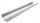How much metal sheet is needed to produce 8 gutters 4 m long and 12 cm in diameter? During production joints calculate at 3% of total consumption.
• GutterHow much metal is needed for production 60 pieces of gutter pipes with the diameter 17 cm and length of 6 m? The plate bends add 6% of the material.
• Barrel 2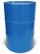Empty barrel weighs 7 kg. When it filled with water up to 45% height, weighs 60 kg. How heavy is barrel full of water?
• Water containerContainer with water weighs 1.48 kg. When we cast 75% of water container of water weight 0.73 kg. How heavy is an empty container?
• Barrel 3Barrel with water has a weight 118 kg. When we get off 75% of water it has a weight 35 kg. How many kg has empty barrel?
• VintnerHow high can vintner fill keg with crushed red grapes if these grapes occupy a volume of 20 percent? Keg is cylindrical with a diameter of the base 1 m and a volume 9.42 hl. Start from the premise that says that fermentation will fill the keg (the number
• Barrel of oilBarrel of oil weighs 283 kg. When it mold 26% oil, weighed 216 kg. What is the mass of the empty barrel?

Do you have an interesting mathematical word problem that you can't solve it? Submit a math problem, and we can try to solve it.

We will send a solution to your e-mail address. Solved examples are also published here. Please enter the e-mail correctly and check whether you don't have a full mailbox.

Please do not submit problems from current active competitions such as Mathematical Olympiad, correspondence seminars etc...

Our percentage calculator will help you quickly calculate various typical tasks with percentages. Percentages Problems. Cylinder Problems.